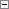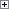This simple data type denotes a special type of string that is converted to uppercase and removes any trailing or leading spaces.Remarks

The length of a Code variable equals the number of characters in the text without leading or trailing spaces.

Microsoft Dynamics NAV 2013 R2 does not enforce a limit on the length of a Code variable. You can specify a maximum length in the C/AL Globals or C/AL Locals window when you create the variable, but it is not required. There is no performance impact if you do not specify a length. The maximum length of a Code field in a table is 250 characters.

A Code variable cannot be null.

The Code data type supports Unicode.

You can index any character position in a string, such as A. The resulting value will be a Char Data Type.

Fields that contain a date formula must not have data type Code. Instead, use the DateFormula Data Type. All fields that contain a date formula with data type Code must be converted into data type DateFormula.

You cannot assign a char to a position in the code variable greater than the current length of the variable +1. For more information, see Char Data Type.Example

This example shows some typical examples of code string assignments. In these examples, assume that the variable c is a code variable with a maximum length of 4.Copy Code
c := 'ABC';
// Results in variable c, which contains 'ABC'
// and is 3 characters in length.
c := '1';
// Results in variable c, which contains '1'
// and is 1 character in length.
c := '';
// Results in variable c, which contains '' (empty string)
// and is zero (0) characters in length.
c := ' 2 ';
// Results in variable c, which contains '2'
// and is 1 character in length.